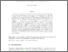# Limit theorems for continuous-state branching processes with immigration

Foucart, Clément, Ma, Chunhua and Yuan, Linglong
(2020) Limit theorems for continuous-state branching processes with immigration. [Preprint]Text cbi-arxiv.pdf - Submitted version Download (532kB) | Preview

## Abstract

We prove and extend some results stated by Mark Pinsky: Limit theorems for continuous state branching processes with immigration [Bull. Amer. Math. Soc. 78(1972), 242--244]. Consider a continuous-state branching process with immigration $(Y_t,t\geq 0)$ with branching mechanism $\Psi$ and immigration mechanism $\Phi$ (CBI$(\Psi,\Phi)$ for short). We shed some light on two different asymptotic regimes occurring when $\int_{0}\frac{\Phi(u)}{|\Psi(u)|}du<\infty$ or $\int_{0}\frac{\Phi(u)}{|\Psi(u)|}du=\infty$. We first observe that when $\int_{0}\frac{\Phi(u)}{|\Psi(u)|}du<\infty$, supercritical CBIs have a growth rate dictated by the branching dynamics, namely there is a renormalization $\tau(t)$, only depending on $\Psi$, such that $(\tau(t)Y_t,t\geq 0)$ converges almost-surely to a finite random variable. When $\int_{0}\frac{\Phi(u)}{|\Psi(u)|}du=\infty$, it is shown that the immigration overwhelms the branching dynamics and that no linear renormalization of the process can exist. Asymptotics in the second regime are studied in details for all non-critical CBI processes via a nonlinear time-dependent renormalization in law. Three regimes of weak convergence are then exhibited, where a misprint in Pinsky's paper is corrected. CBI processes with critical branching mechanisms subject to a regular variation assumption are also studied.

Item Type: Preprint 24 pages math.PR, math.PR, primary 60J80, secondary 60F05, 60F15 Symplectic Admin 05 Oct 2020 07:49 18 Jan 2023 23:30 Author https://livrepository.liverpool.ac.uk/id/eprint/3103433